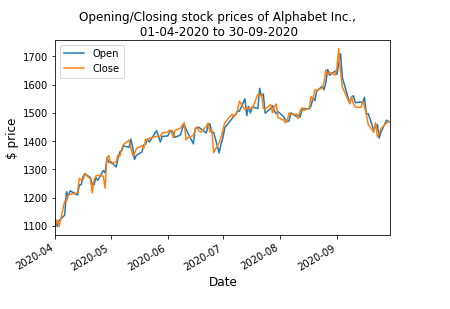﻿ Pandas: Create a line plot of one column versus other columns - w3resource# Pandas: Create a line plot of one column versus other columns

## Pandas: Plotting Exercise-2 with Solution

Write a Pandas program to create a line plot of the opening, closing stock prices of Alphabet Inc. between two specific dates.

Use the alphabet_stock_data.csv file to extract data.

alphabet_stock_data:

alphabet_stock_data Table

Date Open High Low Close Adj Close Volume
2020-04-01 1122 1129.689941 1097.449951 1105.619995 1105.619995 2343100
2020-04-02 1098.26001 1126.859985 1096.400024 1120.839966 1120.839966 1964900
2020-04-03 1119.015015 1123.540039 1079.810059 1097.880005 1097.880005 2313400
2020-04-06 1138 1194.660034 1130.939941 1186.920044 1186.920044 2664700
... ... ... ... ... ... ...
... ... ... ... ... ... ...
2020-09-29 1470.390015 1476.662964 1458.805054 1469.329956 1469.329956 978200
2020-09-30 1466.800049 1489.75 1459.880005 1469.599976 1469.599976 1700600

Sample Solution:

Python Code :

``````import pandas as pd
import matplotlib.pyplot as plt
start_date = pd.to_datetime('2020-4-1')
end_date = pd.to_datetime('2020-09-30')
df['Date'] = pd.to_datetime(df['Date'])
new_df = (df['Date']>= start_date) & (df['Date']<= end_date)
df2 = df.loc[new_df]
plt.figure(figsize=(10,10))
df2.plot(x='Date', y=['Open', 'Close']);
plt.suptitle('Opening/Closing stock prices of Alphabet Inc.,\n 01-04-2020 to 30-09-2020', fontsize=12, color='black')
plt.xlabel("Date",fontsize=12, color='black')
plt.ylabel("\$ price", fontsize=12, color='black')
plt.show()

``````

Sample Output:

``````

Python Code Editor:

Have another way to solve this solution? Contribute your code (and comments) through Disqus.

What is the difficulty level of this exercise?

Test your Python skills with w3resource's quiz

﻿

## Python: Tips of the Day

Trenary operator:

```>>> "Python ROCK" if True else " I AM GRUMPY"
"Python ROCK"
```